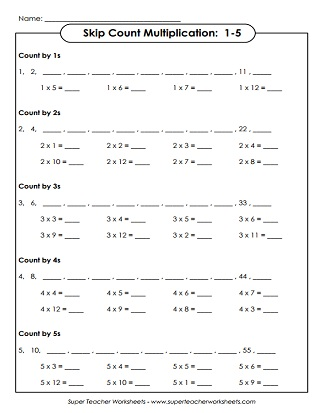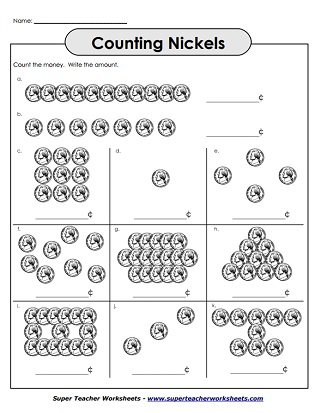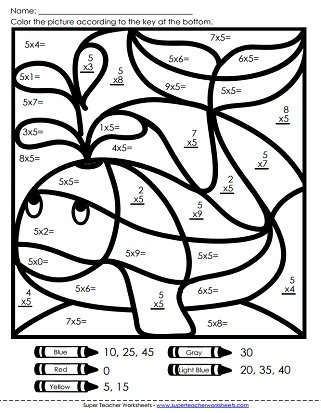# Multiplying by 5s

Here you'll find our collection of worksheets on multiplying by 5s. Includes worksheets with all facts from 0-5, as well as worksheets with only 5s, as well as skip counting by 5s.## Multiplication by5s Only

On this page, students begin with a skip counting activity. Then they complete a mixed-up 5s multiplication table. After that, they'll compare products using the symbols <, >,
and =.
Solve the facts on the page, then color according to the key. The secret picture is a happy blue whale.
This file contains 24 task cards. Questions and problems include arrays, fact triangles, group models, and repeated addition.
Ace your multiplication facts with 5s and 6s with these printables. There are several different activities that can be played with these cut-outs.
Children will multiply by 5s in order to fill out every bubble on the multiplication caterpillar.
Kids can create an origami cootie catcher to review their 5s times tables.
This set contains 16 flash cards. There's also a sorting mat and a practice quiz.
Print this file on thick card stock. Cut out the penguin and the slider strip. Weave the strip with multiplication facts into the penguin. Read each fact and say the answer.

## Skip Counting by 5s

Count the fish, flowers, snails, and crayons. Use knowledge of "counting by five" to help. Goes up to 45.
Answer the word problems and thinking questions by skip counting.
Complete the dot-to-dot. The finished picture is a might elephant.
Fill in the missing numbers on the number line. Count 0, 5, 10, 15, 20, 25, and so on.
This worksheet has a golf-theme. Skip count by 5s up to 120.
Skip count by 5s all the way up to 120. Write answers on the penguins' bodies.
Some of the balloons don't have numbers. Follow the pattern and write the missing numbers on the empty balloons.
Tell how much money is shown by each group of nickels.

## All Facts0s through 5s

This drill worksheet has multiplication tables for 0s, 1s, 2s, 3s, 4s, and 5s. 43 problems. Facts through 10, up to 5x10.
This drill-and-practice page has multiplication tables for 0s, 1s, 2s, 3s, 4s, and 5s. 39 problems total. Facts through 10, up to 5x10, up to 5x12.
The key to mastering fluency is to practice answering facts as quickly and accurately as possible. This timed test can help. Facts through 12, up to 5x12.
First fill in the skip count number lines, then write the answers to the multiplication facts.
Multiplication Basic Facts: 0-10

This page has multiplication worksheets for all basic facts, up to 10x10. (And we also have some that go up to 12x12.)

Multiplication/Division
Fact Families

Fact families are used to teach relationships between division and multiplication.

Multiplication by 3s

The printables on this page can be used to help teach the 3s times tables.

Multiplication by 4s

Multiplying single-digit numbers with 4 as a factor is the topic for this page.

Multiplication by 6s

Multiplication by 7s

Basic facts with factors of 7 are the focus on these printable learning resources.

Multiplication by 8s

Practice multiplying by the number 8. Includes mystery pictures, skip counting lessons, and more.

Multiplication by 9s

## Sample Worksheet ImagesMy Account
Site Information